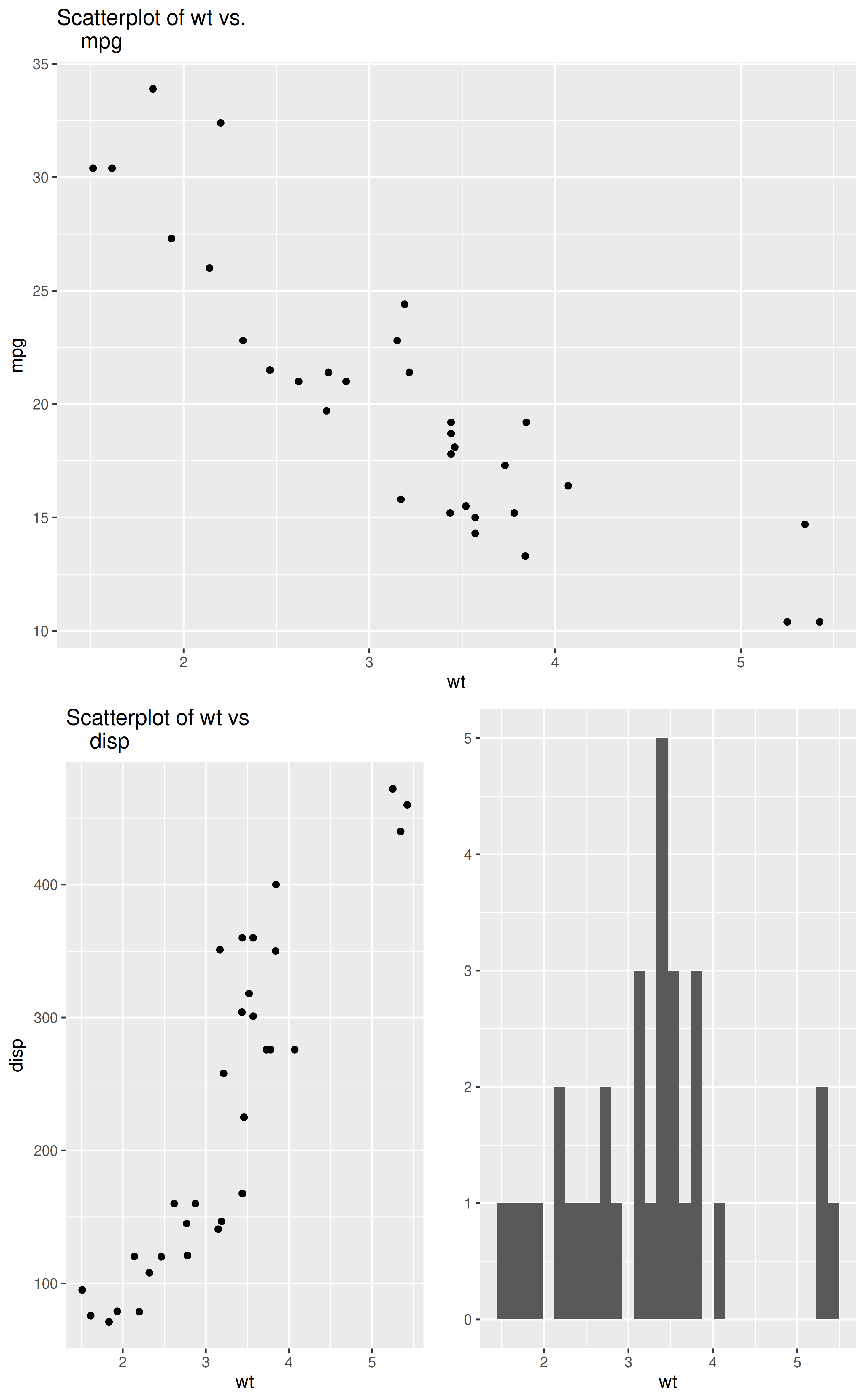Arrange ggplot2 plots in an arbitrary grid

lay_out(...)

## Arguments

... A series lists of of ggplot objects

## Value

A grid of ggplot2 plots

## Details

The function takes arguments of the form list(plot, row(s), column(s)) where plot is a ggplot2 plot object, and the rows and columns identify an area of the grid that you want that plot object to occupy. See http://stackoverflow.com/questions/18427455/multiple-ggplots-of-different-sizes

## Author

Extracted from the [wq] package

## Examples

library(ggplot2)
p1 <- qplot(x=wt,y=mpg,geom="point",main="Scatterplot of wt vs.
mpg"lot(x=wt,y=mpg,geom="point",main="Scatterplot of wt vs.
mpg", data=mtcars)
p2 <- qplot(x=wt,y=disp,geom="point",main="Scatterplot of wt vs
disp"ot(x=wt,y=disp,geom="point",main="Scatterplot of wt vs
disp", data=mtcars)
p3 <- qplot(wt,data=mtcars)
lay_out(list(p1, 1:2, 1:4),
list(p2, 3:4, 1:2),
list(p3, 3:4, 3:4))#> stat_bin() using bins = 30. Pick better value with binwidth.